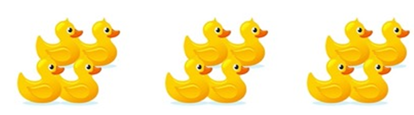Mathematics
Easy

Question

# _______toy ducks are divided equally into _____ groups.## The correct answer is: 12, 3

### Total ducks = 12One group = 4 ducks12 ÷ 4 = 312 ducks are divided into 3 groups.

From the figure, we can count that there are 12 ducks which have been divided equally into 3 groups. Each group consists of 4 ducks each.

### Related Questions to study#### With Turito Foundation.#### Get an Expert Advice From Turito.